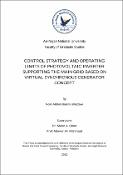## CONTROL STRATEGY AND OPERATING LIMITS OF PHOTOVOLTAIC INVERTER SUPPORTING THE MAIN GRID BASED ON VIRTUAL SYNCHRONOUS GENERATOR CONCEPT2022-04-17
##### Authors
Ghazzawi, Noor Aldeen
##### Publisher
An-Najah National University
##### Abstract
This thesis presents a control scheme of virtual synchronous generator in photovoltaic inverters without using external energy storage systems, where instead the reserve active power is used to improve the grid frequency as well as the grid voltage. The reserve power concept is possible in the smart grid context because some of the power inverters are smart inverters and all are controlled by a control unit. The grid operator can reduce the output power from these inverters in the case the power generated from the inverters is more than the power required by the load. Meanwhile, the active power can be reduced or increased even without using external storage which is with high investment cost. The case study includes a PV inverter with a rated power of 50 kVA, a PV system with a rated power of 50 kW, and a grid voltage of 380 V. When the solar radiation was 200 W/m2, and the load power was 120 kW, 37.5 kVAr, the load voltage was 202.4 V. The proposed control strategy improved the voltage by 17.6 V to be 220V. When the solar radiation was 1000 W/m2, and the load power was 20kW, 7.5kVAr, the load voltage was 230V. The proposed control reduced the voltage by 10 V to be 220 V. When there was a variable frequency of ±0.4 Hz from the nominal frequency of 50 Hz, the proposed control improved the frequency by ±0.4 Hz to be 50 Hz. In the case of low load voltage (204 V) with a rise in system frequency (50.2 Hz), the proposed control strategy improved the voltage by 15V to be 219 V and improved the frequency by reducing the output power of the PV system by 4kW. The MATLAB/SIMULINK software was used to simulate and to show the effectiveness of the proposed control strategy to improve system stability with different scenarios.
##### Description
This thesis presents a control scheme of virtual synchronous generator in photovoltaic inverters without using external energy storage systems, where instead the reserve active power is used to improve the grid frequency as well as the grid voltage. The reserve power concept is possible in the smart grid context because some of the power inverters are smart inverters and all are controlled by a control unit. The grid operator can reduce the output power from these inverters in the case the power generated from the inverters is more than the power required by the load. Meanwhile, the active power can be reduced or increased even without using external storage which is with high investment cost. The case study includes a PV inverter with a rated power of 50 kVA, a PV system with a rated power of 50 kW, and a grid voltage of 380 V. When the solar radiation was 200 W/m2, and the load power was 120 kW, 37.5 kVAr, the load voltage was 202.4 V. The proposed control strategy improved the voltage by 17.6 V to be 220V. When the solar radiation was 1000 W/m2, and the load power was 20kW, 7.5kVAr, the load voltage was 230V. The proposed control reduced the voltage by 10 V to be 220 V. When there was a variable frequency of ±0.4 Hz from the nominal frequency of 50 Hz, the proposed control improved the frequency by ±0.4 Hz to be 50 Hz. In the case of low load voltage (204 V) with a rise in system frequency (50.2 Hz), the proposed control strategy improved the voltage by 15V to be 219 V and improved the frequency by reducing the output power of the PV system by 4kW. The MATLAB/SIMULINK software was used to simulate and to show the effectiveness of the proposed control strategy to improve system stability with different scenarios.
##### Keywords
virtual synchronous generator, distributed generation, high penetration, frequency stability, voltage stability, power reserve control.